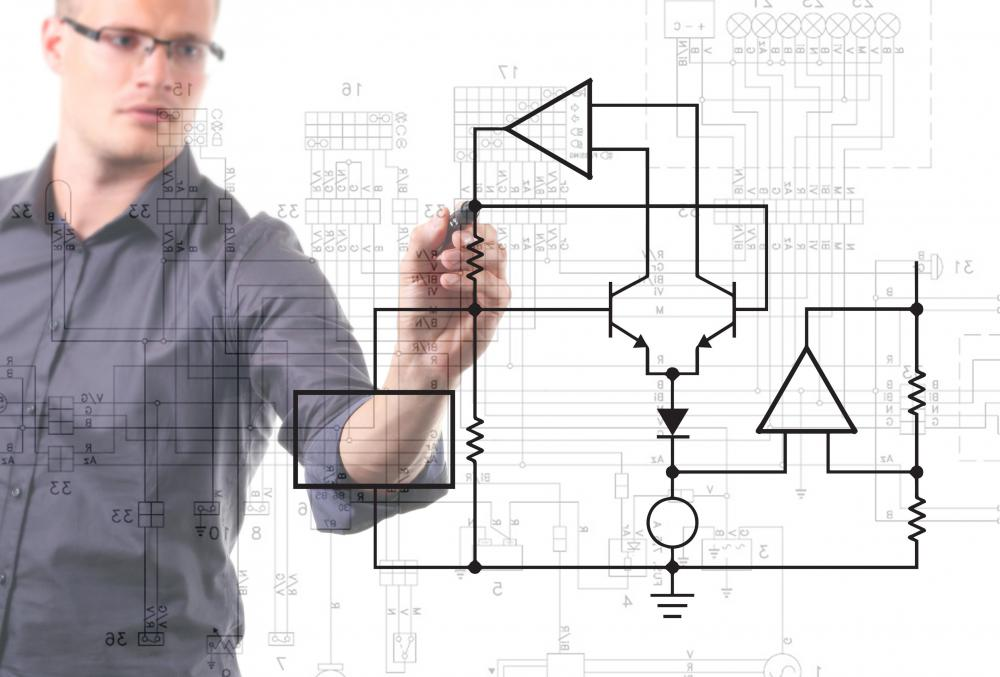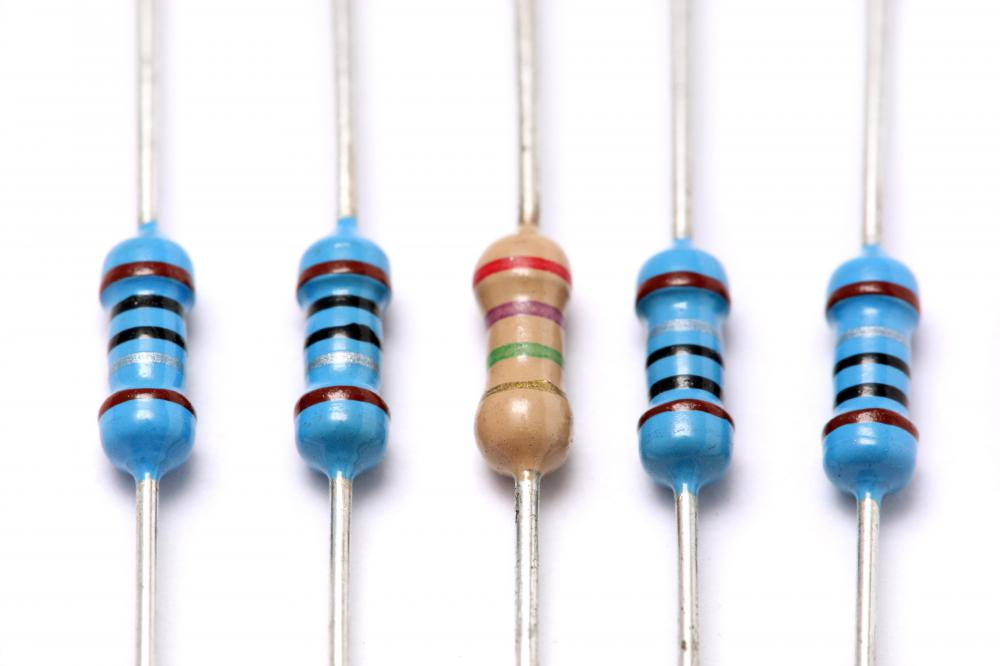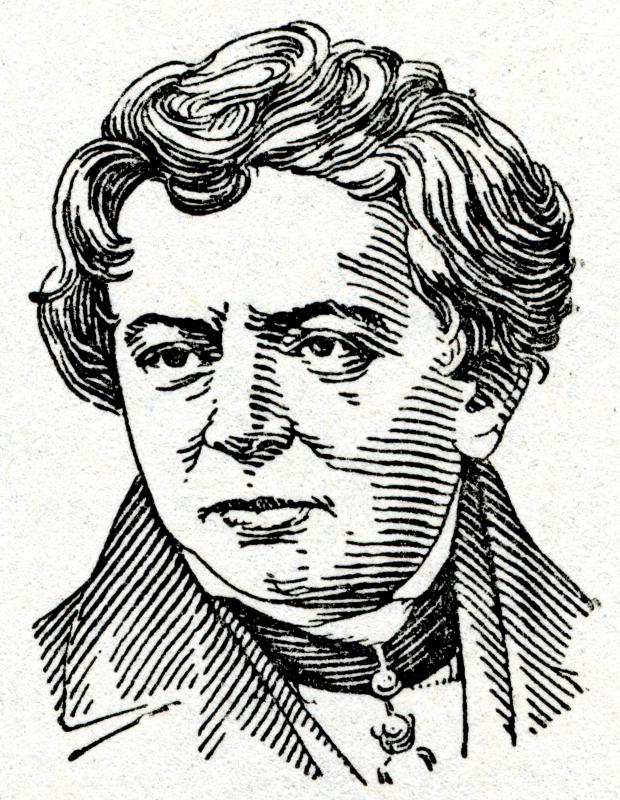# What is an Electrical Circuit?

Rebecca Partington

An electrical circuit is a device that uses electricity to perform a task, such as run a vacuum or power a lamp. The circuit is a closed loop formed by a power source, wires, a fuse, a load, and a switch. Electricity flows through the circuit and is delivered to the object it is powering, such as the vacuum motor or lightbulb, after which the electricity is sent back to the original source; this return of electricity enables the circuit to keep the electricity current flowing. Three types of electrical circuits exist: the series circuit, the parallel circuit, and the series-parallel circuit; depending on the circuit type, it may be possible for electricity to continue flowing should a circuit stop working. Two concepts, Ohm's Law and source voltage, can affect the amount of electricity flowing through a circuit, and therefore, how well an electrical circuit functions.

#### How it Works

Most devices that run on electricity contain an electrical circuit; when connected to a source of power, such as being plugged into an electrical outlet, electricity can run through the electrical circuit within the device and then return to the original power source, to continue the flow of electricity. In other words, when a power switch is turned on, the electrical circuit is complete and current flows from the positive terminal of the power source, through the wire to the load, and finally to the negative terminal. Any device that consumes the energy flowing through a circuit and converts that energy into work is called a load. A light bulb is one example of a load; it consumes the electricity from a circuit and converts it into work — heat and light.

#### Types of Circuits

A series circuit is the simplest because it has only one possible path that the electrical current may flow; if the electrical circuit is broken, none of the load devices will work. The difference with parallel circuits is that they contain more than one path for electricity to flow, so if one of the paths is broken, the other paths will continue to work. A series-parallel circuit, however, is a combination of the first two: it attaches some of the loads to a series circuit and others to parallel circuits. If the series circuit breaks, none of the loads will function, but if one of the parallel circuits breaks, that parallel circuit and the series circuit will stop working, while the other parallel circuits will continue to work.

#### Ohm's Law

Many "laws" apply to electrical circuits, but Ohm's Law is probably the most well known. Ohm's Law states that an electrical circuit's current is directly proportional to its voltage, and inversely proportional to its resistance. So, if voltage increases, for example, the current will also increase, and if resistance increases, current decreases; both situations directly influence the efficiency of electrical circuits. To understand Ohm's Law, it's important to understand the concepts of current, voltage, and resistance: current is the flow of an electric charge, voltage is the force that drives the current in one direction, and resistance is the opposition of an object to having current pass through it. The formula for Ohm's Law is E = I x R, where E = voltage in volts, I = current in amperes, and R = resistance in ohms; this formula can be used to analyze the voltage, current, and resistance of electricity circuits.Amps, volts, watts, and ohms measure different aspects of electricity as it travels through a circuit.

#### Source Voltage

Another important concept in regards to electrical circuits, source voltage refers to the amount of voltage that is produced by the power source and applied to the circuit. In other words, source voltage depends on how much electricity a circuit will receive. Source voltage is affected by the amount of resistance within the electrical circuit; it can also affect the amount of current, as the current is typically affected by both voltage and resistance. Resistance is not affected by voltage or current, however, but can reduce the amounts of both voltage and current to a electrical circuits.Resistors are electrical devices that manage the flow of current through a circuit.German physicist Georg Ohm uncovered how a material's make up, length and thickness influences how much current will flow through it at a certain voltage.

## You might also Likeanon259178

So what's the voltage drop, or current drop, across a series or parallel circuit? This article has the usual textbook stuff fluff but not the stuff I want to know.

anon145091

anon142622

Wow, very helpful. Thanks. Because I needed to know about all this stuff for my science exams. Thank you. Now I think I'm going to pass. Thank you.

anon113335

I found it very interesting, helpful and easy to understand.

anon105543

anon93485

anon89792

It's good. i like it and it was very helpful. Thank you very much.

anon89671

I found it is very helpful.

anon84151

Wow. I've been looking for something like this for so long, and i finally find it straight and easy over here. Really helpful. Thanks bud!

anon83776

good stuff there mate.

anon80745

this really helped me.

anon79374

laser

anon78555

anon75804

anon75136

This was extremely helpful. Thank you.

anon69244

i easily understand my report. now i know what in going to do.

anon67424

It's good. i like it.

anon49620

yes this was easy to understand and it gave me a lot of information to put in my essay about electricity. thank you.

anon47241

anon17842

I found it very helpful and easy to understand.